##### Basic Math & Pre-Algebra All-in-One For Dummies (+ Chapter Quizzes Online)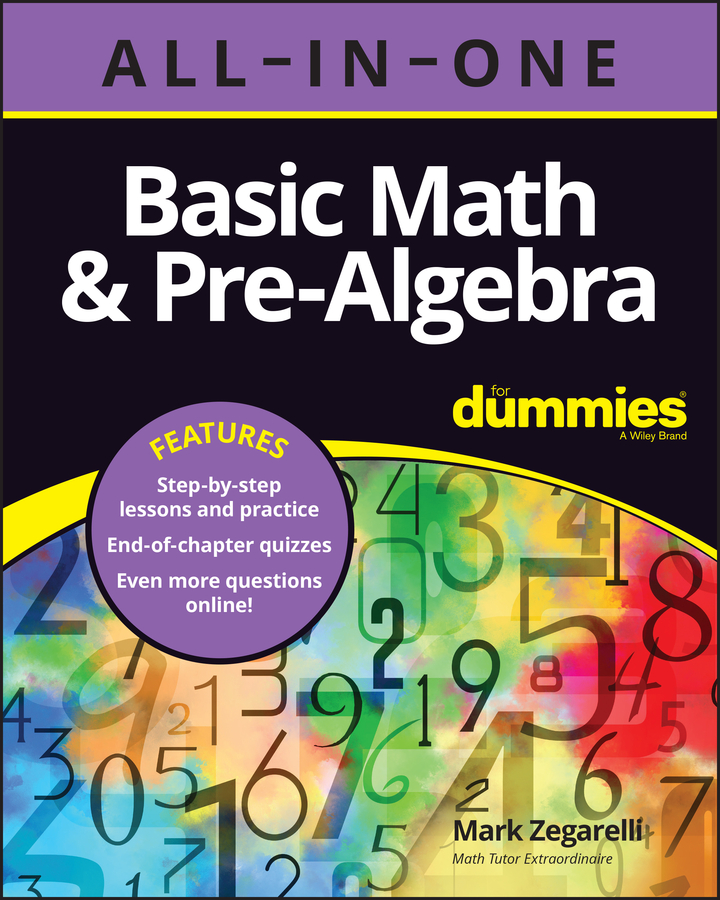The percent circle is a simple visual aid that helps you make sense of percent problems so that you can solve them easily. The three main types of percent problems are finding the ending number, finding the percentage, and finding the starting number.

The trick to using a percent circle is to write information into it. For example, the following figure shows how to record the information that 50% of 2 is 1.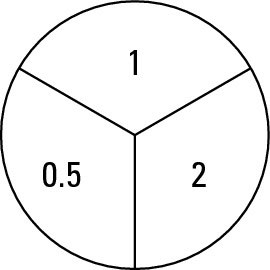Notice that as you fill in the percent circle, you change the percentage, 50%, to its decimal equivalent, 0.5.

Here are the two main features of the percent circle:

• When you multiply the two bottom numbers together, they equal the top number:

0.5 2 = 1

• If you make a fraction out of the top number and either bottom number, that fraction equals the other bottom number: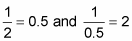These features are the heart and soul of the percent circle. They enable you to solve any of the following three types of percent problems quickly and easily.

Most percent problems give you enough information to fill in two of the three sections of the percent circle. But no matter which two sections you fill in, you can find out the number in the third section.

## Problem type 1: Find the ending number from the percent and starting number

Suppose you want to find out the answer to this problem:

What is 75% of 20?

You’re given the percent and the starting number and asked to find the ending number. To use the percent circle on this problem, fill in the information as shown in the following figure.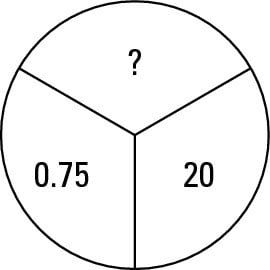Because 0.75 and 20 are both bottom numbers in the circle, multiply them to get the answer: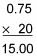So 75% of 20 is 15.

As you can see, this method involves translating the word of as a multiplication sign. You still use multiplication to get your answer, but with the percent circle, you’re less likely to get confused.

## Problem type 2: Find the percentage from the starting and ending numbers

In the second type of problem, you start with both the starting and ending numbers, and you need to find the percentage. Here’s an example:

What percent of 50 is 35?

In this case, the starting number is 50 and the ending number is 35. Set up the problem on the percent circle as shown in the following figure.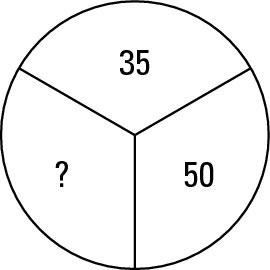This time, 35 is above 50, so make a fraction out of these two numbers:This fraction is your answer, and all you have to do is convert the fraction to a percent. First, convert 35/50 to a decimal: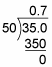Now convert 0.7 to a percent:

0.7 = 70%

## Problem type 3: Find the starting number from the percentage and ending number

In the third type of problem, you get the percentage and the ending number, and you have to find the starting number. For example,

15% of what number is 18?

This time, the percentage is 15% and the ending number is 18, so fill in the percent circle as shown in the following figure.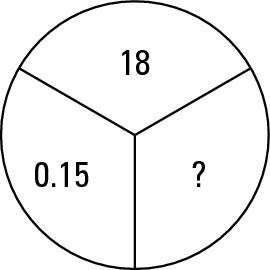Because 18 is above 0.15 in the circle, make a fraction out of these two numbers: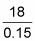This fraction is your answer; you just need to convert to a decimal: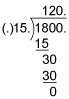In this case, the “decimal” you find is the whole number 120, so 15% of 120 is 18.